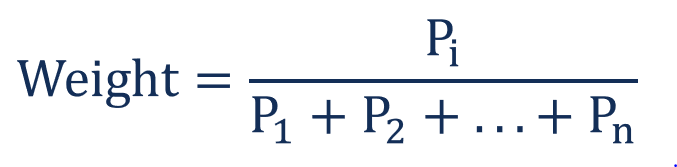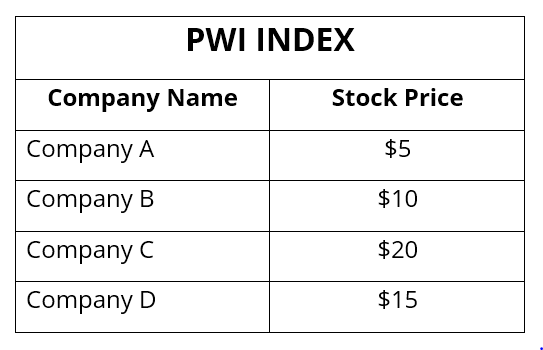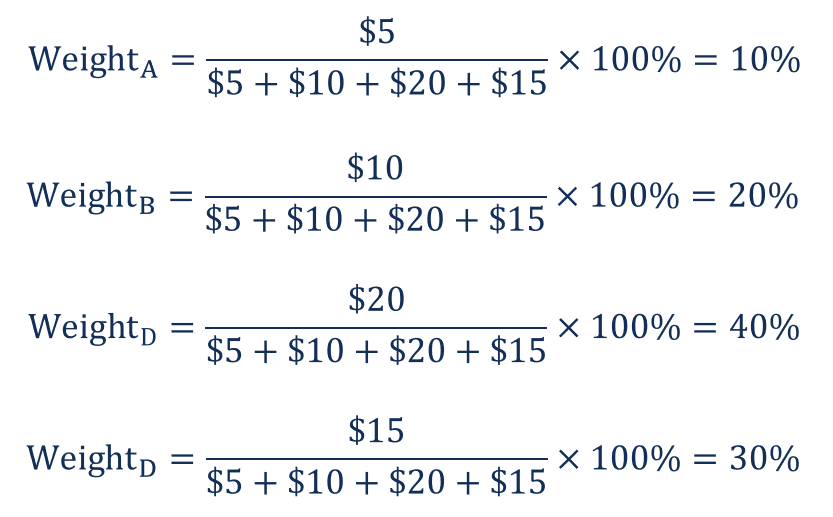# Price-Weighted Index

A stock market index wherein each component is weighted according to its current share price

## What is the Price-Weighted Index?

A price-weighted index is a type of stock market index in which each component of the index is weighted according to its current share price. In price-weighted indices, companies with a high share price have a greater weight than those with a low share price. Therefore, the price movements of companies with the highest share price have the largest impact on the value of the index.

Nowadays, price-weighted indices are less common than other indices. Instead, the capitalization-weighted index is the most prevalent form of market indices. The biggest price-weighted indices are the US Dow Jones Industrial Average (DJIA) and Japan’s Nikkei 225.A price-weighted index is often criticized because it considers only the price of each component as the driver of the index value. Therefore, even a small price fluctuation in a higher priced company may significantly affect the value of the index.

### How to Calculate the Weights in a Price-Weighted Index

The weight of a individual component is calculated by dividing its price by the sum of all the components’ prices. Mathematically, it is expressed in the following way:Let’s consider the following example. The PWI Index is a price-weighted index that includes the stocks of four companies. The information about the companies included in the index can be found in the table below:Using the formula above, we can calculate the weight of each index component:### How to Calculate the Value of a Price-Weighted Index

In theory, the value of the index can be determined as an arithmetic average by dividing the total sum of the prices of the components in the index by the number of the index components. However, such an approach is not usually encouraged because events such as spinoffs, stock splits, and mergers affect the composition of the index.

In reality, the value of a price-weighted index is calculated by dividing the total sum of the prices of the index components by the divisor. The divisor is an arbitrary value computed by the index and adjusted for various structural changes in the index components.

For example, the Dow Jones Industrial Average, which is the most prominent price-weighted index, calculates its own divisor (Dow divisor). The Dow divisor changes over time to better match the existing composition of the index.

### More Resources

CFI is the official provider of the global Financial Modeling & Valuation Analyst (FMVA)™ certification program, designed to help anyone become a world-class financial analyst. To keep advancing your career, the additional resources below will be useful:

• New York Stock Exchange (NYSE)
• Technical Analysis: A Beginner’s Guide